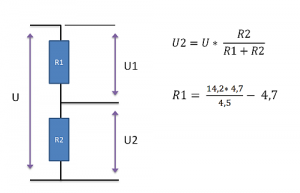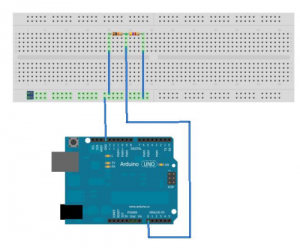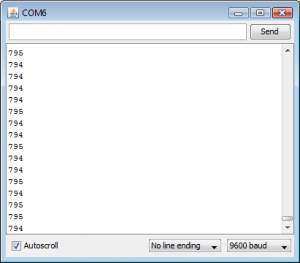# Voltage Divider project

Most mobile robots have batteries to drive them, even the noisy ones powered by an engine. You have to make sure not to discharge you battery below a certain voltage to protect them from damaging. In this project we going to measure the voltage of the batteries with use of an analog port of an Arduino One and the principle of Voltage dividing.

In my case I have a NiMh Battery with 10 Cells. Those 10 Cells are rated at 12V. When fully charged this battery pack has 14,2 V as a maximum.
To protect the battery pack I don’t want the Voltage to drop below 10V. The Arduino analog input has a range from 0V – 5V. The 14,2 Volt is to high too connect directly to the analog input. With two resistors we can produce the needed voltage.

To determine which resistors we need we use the diagram and the formula below. The U is the battery voltage. The resistors R1 and R2 have respectively voltage U1 and U2.U is 14,2V and U2 has to be 5V maximum. I like to be safe so I keep U2 at 4,5V. We still have two unknown values. The use of resistors means power dissipation and therefore we have low values. But if the resistance is to low the analog input of the Arduino will not work. The 4,7 kOhm is a good starting point for the R2 resistor so we use this in the formula.

The result of the formula will be around 10,13 kOhm. We use a 10 KOhm resistor.

R1 = 10kOhm = 10000 Ohm (brown, Black, Orange)
R2 = 4,7kOhm = 4700 Ohm (Yellow, purple, red)

To do check which color coding your need, check https://swanrobotics.com/tools/resistorcolorcoding/ for the right values.

Electronics
The picture below shows the breadboard that I used and was done with http://Fritzing.org .Arduino program

[code lang=”cpp”]
void setup() {
Serial.begin(9600);
}

</span>void loop() {
Serial.println(sensorValue);
}
[/code]The Arduino gives numbers instead of the right Voltage so we have to calculate it before we can display it. First we need to know which value represent which value. So simple measure the Voltage and also see what the value is on the screen.

940 = 14,2 Volt
794 = 12 Volt
662 = 10 Volt